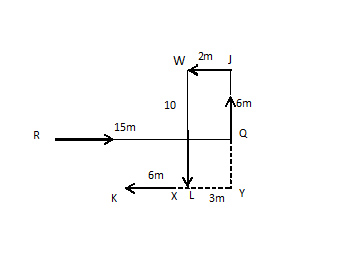# Myntra Reasoning Question

DIRECTIONS for questions 1-3:Study the given information carefully to answer the given question.
R is 15m west of Q. J is 6m north of Q. W is 2m west of J. L is 10m south of W. K is 6m west of L.
1. If F is 4m to the south of R and V is 2m east of K, how far is Point F from Point V?
1. 8m
2. 11m
3. 5m
4. 9m
5. 4mThe following diagram can be formed with the given information.
Now, FL = RQ – WJ = 15 – 2 = 13m
Further, FV = FL – VL = 13 – 4 = 9m
1. Kabir walks 10m towards south from Point J, takes a right turn and walks for 3m. How far will he be from Point K?
1. 4m
2. 10m
3. 9m
4. 6m
5. 5mAs shown in the diagram, if Kabir walks 10 m towards south from point J, he will reach at point Y. Then, moving 3 m to the right side, he will finally reach point X.
Now, XL = XY – LY = 3 – 2 = 1 m
And distance between X and K = KL – XL = 6 – 1 = 5m
2. In which direction is R with respect to J?
1. West
2. South east
3. North east
4. North
5. South westAs per the diagram, it can be observed that R is towards South-west with respect to J..

DIRECTIONS for questions 4-8: Study the following information to answer the following question.
Nine persons- G, H, I, J, K, L, R, T and U are seated in a straight line facing North, with equal distance between each other but not necessarily in the same order.

Only two people sit to the left of L. Only one person sits between I and U. H sits fourth to the right of R. R is not an immediate neighbor of U. Less than three people sit between R and U. Number of people sitting between I and U is half as that between H and J. Only three people sit between K and T. K is not an immediate neighbor of J. Only two people sit between K and G.
3. In which of the given pairs of people, is odd number of people sitting between them?
1. H, T
2. I, H
3. U, R
4. J, K
5. G, K
The following arrangement can be made with the help of given information:
G R L K U H I T J
As we can observe, only option 1 has odd number of persons i.e.,
one person sitting between H and T. Rest all the options have an even number of persons sitting between them.
4. Which of the following is true with respect to L as per the given arrangement?
1. L is an immediate neighbor of U.
2. None of the given options is true.
3. L sits at one of the extreme ends of the line.
4. More than two people sit between L and R.
5. L sits second to the left of G.
The following arrangement can be made with the help of given information:
G R L K U H I T J
From the above arrangement, it can be observed that none of the given options is true.
Hence, option 2 is correct
5. Who amongst the following sit exactly between T and G?
1. I, J
2. H, J
3. K, U
4. H, S
5. J, R
The following arrangement can be made with the help of given information: G R L K U H I T J Observing from the arrangement, it can be seen that K and U sit exactly between T and G.
6. Who sits second to the left of J?
1. T
2. No one as J sits at one of the extreme ends of the line
3. I
4. G
5. S
The following arrangement can be made with the help of given information: G R L K U H I T J I sits second to the left of J.
7. Which two persons are sitting at the two ends of the sitting arrangement?
1. R, T
2. R, J
3. R, I
4. H, G
5. J, G
The following arrangement can be made with the help of given information: G R L K U H I T J It can be observed from the arrangement that J and G are sitting at two ends of the row.
DIRECTIONS for questions 9-10: What should come in place of question mark (?) in the following number/alphabetic series?
1. Only conclusion I is true
2. Only conclusion II is true
3. Either conclusion I or II is true
4. Both conclusions I and II are true
5. Neither conclusion I nor II is true

8. Statements:M ≤ A ≥ N; E ≤ A < G

Conclusion I:M ≤ E      Conclusion II:G > N